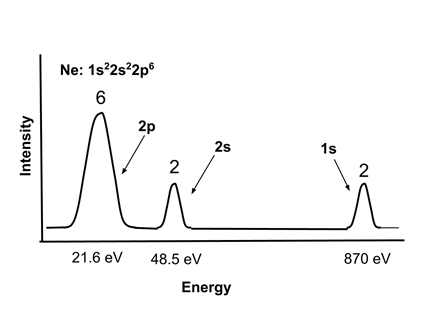# Enduring Understanding 1.B: Atomic Structure and Periodicity

• The properties of atoms arise from the interactions between their nuclei and electrons.

• Atoms are composed of:

• A positively charged nucleus, composed of positively charged protons and neutral neutrons
• Negatively charged electrons that orbit around the nucleus. Electrons can be easily added to or removed from most atoms.

• According to Coulomb's Law, like charges repel each other and unlike charges attract each other. The higher the charge, the greater the attraction/repulsion, and the greater the distance between the charges, the less the attraction/repulsion.
• Therefore, the properties of atoms can be explained by opposite charges (e.g. positive protons and negative electrons) attracting each other, and like charges (e.g. two electrons) repelling each other.

• In an atom, electrons arrange themselves into shells, subshells, and orbitals.
• Each orbital can contain up to two electrons
• S subshells contain one orbital (up to 2 electrons), P subshells contain three orbitals (up to 6 electrons), D subshells contain five orbitals (up to 10 electrons). Larger subshells (F, G...) are rarely used in introductory chemistry.

• Electron Configuration: In order of increasing energy in multielectron atoms, subshells are:
• 1s < 2s < 2p < 3s < 3p < 4s < 4d < 4p < 5s
• Lower energy shells and subshells fill first, so the electron configuration of atoms and ions can be written. Examples:
• Hydrogen, H (1 electron): 1s1
• Helium, He (2 electrons): 1s2
• Lithium, Li (3 electrons): 1s22s1
• Boron, B (5 electrons): 1s22s22p1
• Sodium, Na (11 electrons): 1s22s22p63s1
• When a shell is filled with electrons, this is called a 'noble gas' electron configuration. Noble gas configurations are very stable.
• Filled shells are called core electrons and are very tightly bound to the atom. E.g. in Na, 1s22s22p63s1 can be written as [Ne] 3s1, and the 1s, 2s, and 2p electrons are tightly bound.
• Electrons in the outermost shell are called valence electrons. They are shielded from the nuclear charge by the core electrons. In Na, the 3s1 electron is much more easily removed than the core electrons.

• Ionization energy is the energy required to remove an electron from an atom or ion. It is different for every electron in every ion.
• As mentioned above, valence electrons are easier to remove (have a lower ionization energy) than core electrons.
• Na → Na1+ (3s valence electron) EI1 = 496 kJ/mol
• Na1+ → Na2+ (2p core electron) EI2 = 4560 kJ/mol, nearly 10x higher than the EI1
• In general, first ionization energies:
• Increase going up the periodic table, because electrons in lower shells are closer to the nucleus and less repelled by other electrons, e.g.:
• Li EI1 = 520 kJ/mol, Na EI1 = 496 kJ/mol
• Increase going right across the periodic table, because effective nuclear charge (charge felt by valence electrons) increases across a given row of the periodic table, e.g.:
• C EI1 = 1087 kJ/mol, N EI1 = 1402 kJ/mol
• Exception: Filled and half-filled subshells are somewhat stable, so removing the first electron in a subshell or the first paired electron in a subshell can be lower in energy than from a filled subshell, e.g.:
• O, 1s22s22p4, has two electrons in one of its p orbitals. Due to electron-electron repulsions, removing this electron requires less energy (EI1 = 1314 kJ/mol) than removing an electron from N, 1s22s22p3, (EI1 = 1402 kJ/mol) even though O is right of N in the second row of the periodic table.
• B, 1s22s22p1, has only one electron in its p subshell. Removing this electron requires less energy (EI1 = 801 kJ/mol) than removing an electron from Be, 1s22s2, (EI1 = 900 kJ/mol) since the latter has a filled s subshell.

• Electron energies in atoms can be observed experimentally with Photoelectron Spectroscopy, in which the atoms are bombarded with x-rays and the energy of the ejected electrons measured. The energy of the ejected electrons indicates their energy level, and the intensity of the signal indicates the number of electrons in that energy level in the atom.
• A typical photoelectron spectrum for neon, Ne, 1s22s22p6, is shown. Note that the core 1s electrons are very strongly bound, and the valence 2s electrons are somewhat more tightly bound than the 2p electrons.
• <• Example: An atom has the electron configuration 1s22s22p63s2. Which successive ionization energy will be significantly higher than the one preceding it?
• This electron configuration corresponds to magnesium (Mg). It has two valence electrons, so they should be relatively easy to remove. The third ionization would remove a core 2p electron, and would be expected to be much higher. This is what is observed; the first, second and third ionization energies for Mg are 738, 1451, and 7733 kJ/mol respectively.

 Related Links: Chemistry Chemistry Quizzes AP Chemistry Notes Chemical Analysis AP Chemistry Quizzes Atoms, Molecules, and Chemical Analysis Quiz Electronic Structure of Atoms Quiz Periodicity, Atomic & Ionic Radii, Atomic Models Quiz Conservation of Matter and Balanced Equations Quiz Gases and Solutions Quiz Intermolecular Forces Quiz Molecular Geometry and VSEPR Quiz Bonding and Solid State Properties Quiz Balanced and Ionic Equations Quiz Chemical Reactions Quiz Redox Reactions and Electrochemistry Quiz Chemical Kinetics and Rates Quiz Reaction Rates and Collisions Quiz Multi-Step Reaction Rates and Catalysis Quiz Energy and Heat Quiz Conservation of Energy and Calorimetry Quiz Bond Energies Quiz Entropy and Gibbs Free Energy Quiz Chemical Equilibrium Quiz Solubility and Acid-Base Equilibrium Quiz

To link to this Atomic Structure and Periodicity page, copy the following code to your site: